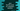# Java program to find the roots of a quadratic equation## Java program to find the roots of a quadratic equation:

In this post, we will learn how to find the roots of a quadratic equation in Java. We will take the values as inputs from the user and print the root values.

### How to find the roots of a quadratic equation:

Below is the formula of a quadratic equation:

``ax^2 + bx + c = 0``

Here, a, b and c are all real numbers and a can’t be equal to 0. We can use the below formula to find the roots of this quadratic equation:

``-b ± √(b^2-4ac)/ 2a``

There will be two roots. The ± sign is used for that.

Also, the value under the square root, b2-4ac is called the discriminant. Based on the value of this discriminant, the roots of a quadratic equation is defined.

• If it is equal to 0, roots are equal and both are real numbers.
• If it is greater than 0, roots are different and both are real numbers.
• If it is less than 0, roots are different and both are complex numbers.

### Java program:

We will take the values of a, b and c as inputs from the user and print the roots for that quadratic equation.

``````import java.util.Scanner;

class Main {

public static void main(String[] args) {
double a, b, c, d;
double firstRoot, secondRoot;

Scanner sc = new Scanner(System.in);

System.out.println("Enter the values of a, b and c: ");
a = sc.nextDouble();
b = sc.nextDouble();
c = sc.nextDouble();

d = b * b - 4 * a * c;

if (d == 0) {
firstRoot = secondRoot = -b / (2 * a);
System.out.format("First root: %.2f, second root: %.2f", firstRoot, secondRoot);
} else if (d > 0) {
firstRoot = -b + Math.sqrt(d) / 2 * a;
secondRoot = -b - Math.sqrt(d) / 2 * a;

System.out.format("First root: %.2f, second root: %.2f", firstRoot, secondRoot);
} else {
double r = -b / (2 * a);
double i = Math.sqrt(-d) / (2 * a);

System.out.format("First root: %.2f+%.2fi\n", r, i);
System.out.format("Second root: %.2f-%.2fi", r, i);

}

}
}``````

Here,

• a, b, c variables are to hold the a, b, and c values of the quadratic equation.
• d is to hold the discriminant.
• We are taking the values of a, b, and c from user and it finds the values of d by using the above formula.
• Based on the value of d, it finds the roots of the quadratic equation.
• The if block will run if the value of d is equal to 0.
• The else if will run if it is greater than 0.
• The else block will run if it is less than 0.

• Inside the blocks, we are finding the roots by using the same formula we seen above. We are using System.out.format to print the values of the roots.

### Sample output:

If you run this program, it will give output as like below:

``````Enter the values of a, b and c:
3
4
5
First root: -0.67+1.11i
Second root: -0.67-1.11i

Enter the values of a, b and c:
1
20
2
First root: -10.10, second root: -29.90``````